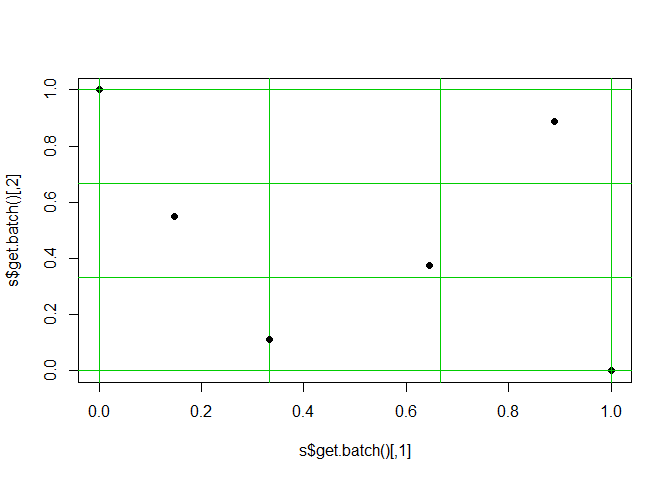# sFFLHD

This R package provides a class that generates experiment sFFLHD designs. Sequential full factorial-based Latin hypercube design were created by Duan, Ankenman, Sanchez, and Sanchez (2015, Technometrics).

To create a new design you use the function `sFFLHD\$new` and must give in the number of dimensions, `D`, and the batch size/number of levels per factor, `L`. An example is shown below (the last line can be repeated when run in console to see how new batches are added).

``````library(sFFLHD)
#>
#> Attaching package: 'DoE.base'
#> The following objects are masked from 'package:stats':
#>
#>     aov, lm
#> The following object is masked from 'package:graphics':
#>
#>     plot.design
#> The following object is masked from 'package:base':
#>
#>     lengths
set.seed(0)
s <- sFFLHD\$new(D=2,L=3)
plot(s\$get.batch(),xlim=0:1,ylim=0:1,pch=19)
abline(h=(0:(s\$Lb))/s\$Lb,v=(0:(s\$Lb))/s\$Lb,col=3);points(s\$get.batch(),pch=19)``````By default the new points are selected using maximin distance optimization to spread them out. This is why points will end up near corners. This option will slow down the code a little but generally not noticeably compared to what the design is used for. If set to `FALSE` then the points are randomly placed within their small grid box.